Courses

# Waves NAT Level - 2

## 10 Questions MCQ Test Topic wise Tests for IIT JAM Physics | Waves NAT Level - 2

Description
This mock test of Waves NAT Level - 2 for Physics helps you for every Physics entrance exam. This contains 10 Multiple Choice Questions for Physics Waves NAT Level - 2 (mcq) to study with solutions a complete question bank. The solved questions answers in this Waves NAT Level - 2 quiz give you a good mix of easy questions and tough questions. Physics students definitely take this Waves NAT Level - 2 exercise for a better result in the exam. You can find other Waves NAT Level - 2 extra questions, long questions & short questions for Physics on EduRev as well by searching above.
*Answer can only contain numeric values
QUESTION: 1

### A observer is standing 10 m from a road on which a vehicle is approaching him at a speed of 96 /hr when the vehicle is 50 m away from him, it blows a horn of frequency 8000 Hz what will be the frequency observed by the observer?

Solution: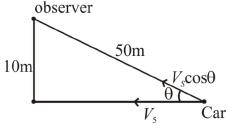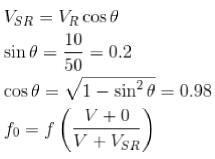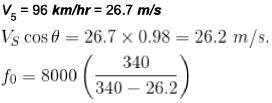= 8668 Hz

*Answer can only contain numeric values
QUESTION: 2

### Consider a pipe 12 cm in length. Find by how much percentage does the fundamental frequency change when the pipe which was originally open at both ends is now closed from one side.

Solution:

Exactly half wavelength fits the pipe,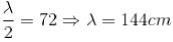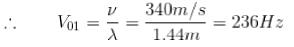When one end is closed then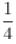of a wavelength fits the pipe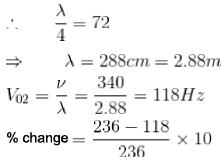= 50%

*Answer can only contain numeric values
QUESTION: 3

### In extremely, cold places, the temperatures are slow that it effects the speed of sound in air. On a day with temperature -35°C, a source emits sound at a frequency of 1050 Hz & moves away from an observer at 25 m/s, the frequency that the observer measures is 97141 Hz. Find the speed of sound (in m/s).

Solution: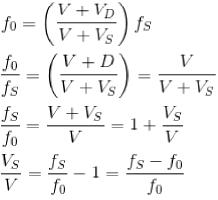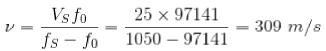*Answer can only contain numeric values
QUESTION: 4

An organ pipe, closed at one and open at the other, is designed to have a fundamental frequency of c( 131 Hz) what is the frequency of the next higher harmonic for this pipe?

Solution:

For a pipe of length L, Closed at one and open at the other end, the resonant frequencies are given by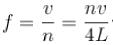where n - 1, 3, 5
and v is the speed of the sound
Thus, a fundamental frequency is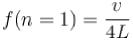and the next harmonic has a frequency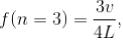For the next harmonic,
f(n = 3) = 3f(n = 1) = 3 x 131
= 393 Hz

*Answer can only contain numeric values
QUESTION: 5

At 20°C, a pipe open at both ends resonates at a frequency of 440 Hz. At what frequency does the same pipe resonate on a cold day when the speed of sounds is 3 percent lower than it would be at 20°C

Solution: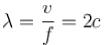Since, 2L remains constant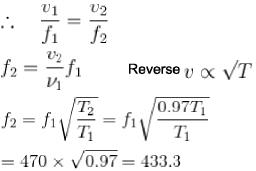*Answer can only contain numeric values
QUESTION: 6

The equation of a wave traveling on a string is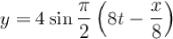.  If x and y are in cm, then velocity of wave is ________ (in cm/s).

Solution:

Direction positive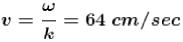*Answer can only contain numeric values
QUESTION: 7

A distant galaxy is observed to have its hydrogen-β line shifted to a wavelength of 580 nm find the approximate velocity of recession of the distant galaxy in unit of 108 m/s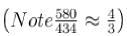Solution:

Applying the relativistic doppler effect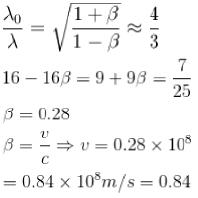*Answer can only contain numeric values
QUESTION: 8

Sound wave moving at 350 m/s difference out of a speaker enclosure with an opening that is a long rectangular slit 0.14m across. At about what frequency will the sound first disappear at an angle of 45° from the normal to the speaker face?

Solution:

The first minimum is determined by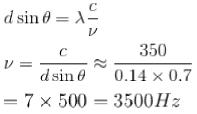*Answer can only contain numeric values
QUESTION: 9

A source is emitting sound with frequency 4371 Hz. Find the magnitude of difference of the two velocities with which the observer must move to observe a frequency that is 20% higher and 20% lower than the actual frequency (in m/s).

Solution:

fs = frequency of source = 4371 Hz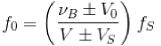When ovserved frequency is 20% higher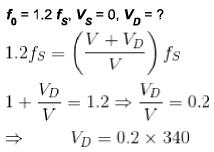= 68 m/s
When the observed frequency is 20% lower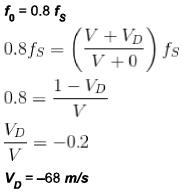(-) sign indicates that the observer is moving away from the source?
∴ Difference of velocities = 68 - (-68)
= 136 m/s

*Answer can only contain numeric values
QUESTION: 10

During a Hurricane, a 1200 Hz warning siren in the town hall sounds the wind is blowing at 55 m/s in a direction from the siren towards a person 1 km away. With what frequency does the sound wave reach the person. The speed of sound in air in 330 m/s.

Solution:

Doppler affect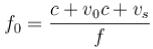C - speed of sound in medium
V0, V5 - Speeds of the observer & source relative to the medium
Since both the source & Since both the source & observer move at the speed of the wind
∴ These is no relative motion between them
No doppler effect
∴ f= 1200 Hz